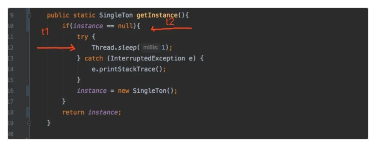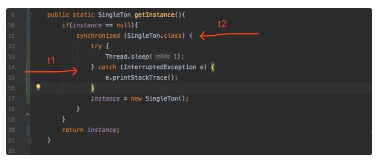#8 种单例模式写法，助你搞定面试！

0
0
01. 云栖社区>
2. 云栖号资讯>
3. 博客>
4. 正文

## 8 种单例模式写法，助你搞定面试！

1. 单例模式常见问题

1.单例类如何控制其实例化
2.如何确保只有一个实例

private构造函数,类的实例话不对外开放,由自己内部来完成这个操作,确保永远不会从类外部实例化类,避免外部随意new出来新的实例。

2. 单例类有哪些特点 ?

3. 单例模式8种写法

1.在多线程环境下行为是否线程安全
2.饿汉以及懒汉
3.编码是否优雅(理解起来是否比较直观)

1. 饿汉式线程安全的

public class SingleTon{

private static final SingleTon INSTANCE = new SingleTon();

private SingleTon(){ }

public static SingleTon getInstance(){
return INSTANCE;
}
public static void main(String[] args) {
SingleTon instance1 = SingleTon.getInstance();
SingleTon instance2 = SingleTon.getInstance();
System.out.println(instance1 == instance2);
}

}

2. 饿汉式线程安全(变种写法)。

public class SingleTon{

private static final SingleTon INSTANCE ;

static {
INSTANCE = new SingleTon();
}

private SingleTon(){}

public static SingleTon getInstance(){
return INSTANCE;
}
public static void main(String[] args) {
SingleTon instance1 = SingleTon.getInstance();
SingleTon instance2 = SingleTon.getInstance();
System.out.println(instance1 == instance2);
}

}

3. 懒汉式线程不安全。

public class SingleTon{

private static  SingleTon instance ;

private SingleTon(){}

public static SingleTon getInstance(){
if(instance == null){
instance = new SingleTon();
}
return instance;
}

public static void main(String[] args) {
SingleTon instance1 = SingleTon.getInstance();
SingleTon instance2 = SingleTon.getInstance();
System.out.println(instance1 == instance2);

// 通过开启100个线程 比较是否是相同对象
for(int i=0;i<100;i++){
System.out.println(SingleTon.getInstance().hashCode())
).start();
}

}

}

// 通过开启100个线程 比较是否是相同对象
for(int i=0;i<100;i++){
System.out.println(SingleTon.getInstance().hashCode())
).start();
}

public static SingleTon getInstance(){
if(instance == null){
try {
} catch (InterruptedException e) {
e.printStackTrace();
}
instance = new SingleTon();
}
return instance;
}4. 懒汉式线程安全(粗粒度Synchronized)。

public class SingleTon{

private static  SingleTon instance ;

private SingleTon(){}

public static SingleTon synchronized getInstance(){
if(instance == null){
instance = new SingleTon();
}
return instance;
}

public static void main(String[] args) {
SingleTon instance1 = SingleTon.getInstance();
SingleTon instance2 = SingleTon.getInstance();
System.out.println(instance1 == instance2);
// 通过开启100个线程 比较是否是相同对象
for(int i=0;i<100;i++){
System.out.println(SingleTon.getInstance().hashCode())
).start();
}

}

}

5. 懒汉式线程不安全(synchronized代码块)

public class SingleTon{

private static  SingleTon instance ;

private SingleTon(){}

public static SingleTon getInstance(){
if(insatnce == null){
synchronied(SingleTon.class){
instance = new SingleTon();
}
}
return instance;
}

public static void main(String[] args) {
SingleTon instance1 = SingleTon.getInstance();
SingleTon instance2 = SingleTon.getInstance();
System.out.println(instance1 == instance2);

// 通过开启100个线程 比较是否是相同对象
for(int i=0;i<100;i++){
System.out.println(SingleTon.getInstance().hashCode())
).start();
}

}

}6. 懒汉式线程安全(双重检验加锁)

public class SingleTon{

private static  volatile SingleTon instance ;

private SingleTon(){}

public static SingleTon getInstance(){
if(instance == null){
synchronied(SingleTon.class){
if(instance == null){
instance = new SingleTon();
}
}
}
return instance;
}

public static void main(String[] args) {
SingleTon instance1 = SingleTon.getInstance();
SingleTon instance2 = SingleTon.getInstance();
System.out.println(instance1 == instance2);

// 通过开启100个线程 比较是否是相同对象
for(int i=0;i<100;i++){
System.out.println(SingleTon.getInstance().hashCode())
).start();
}

}

}

1.分配内存
2.初始化构造器
3.将对象指向分配的内存的地址

7. 静态内部类的方式(基本完美了)

public class SingleTon{

public static SingleTon getInstance(){
return StaticSingleTon.instance;
}
private static class StaticSingleTon{
private static final SingleTon instance = new SingleTon();
}
public static void main(String[] args) {
SingleTon instance1 = SingleTon.getInstance();
SingleTon instance2 = SingleTon.getInstance();
System.out.println(instance1 == instance2);

// 通过开启100个线程 比较是否是相同对象
for(int i=0;i<100;i++){
System.out.println(SingleTon.getInstance().hashCode())
).start();
}

}

}
• 因为一个类的静态属性只会在第一次加载类时初始化，这是JVM帮我们保证的，所以我们无需担心并发访问的问题。所以在初始化进行一半的时候，别的线程是无法使用的，因为JVM会帮我们强行同步这个过程。
• 另外由于静态变量只初始化一次，所以singleton仍然是单例的。

8. 枚举类型的单例模式(太完美以至于。。。)

public Enum SingleTon{

INSTANCE;
public static void main(String[] args) {
// 通过开启100个线程 比较是否是相同对象
for(int i=0;i<100;i++){
System.out.println(SingleTon.getInstance().hashCode())
).start();
}

}

}

【云栖号在线课堂】每天都有产品技术专家分享！

【云栖号在线课堂 社群】https://c.tb.cn/F3.Z8gvnK

+ 关注